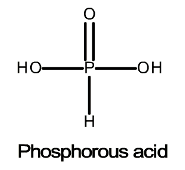# Problem: Phosphorous acid, H3PO4(aq), is a diprotic oxyacid that is an important compound in industry and agriculture.pKa1 = 1.30pKa2 = 6.70Calculate the pH for each of the following points in the titration of 50.0 mL of a 1.5 M H3PO3(aq) with 1.5 M KOH(aq).Part D. after addition of 75.0 mL of KOH Number

###### FREE Expert Solution

Part C. After addition 75.0 mL of KOH

Calculate the volume needed to reach the equivalence point:

VB = 50.0 mL

84% (413 ratings)###### Problem Details

Phosphorous acid, H3PO4(aq), is a diprotic oxyacid that is an important compound in industry and agriculture.pKa1 = 1.30
pKa2 = 6.70

Calculate the pH for each of the following points in the titration of 50.0 mL of a 1.5 M H3PO3(aq) with 1.5 M KOH(aq).

Part D. after addition of 75.0 mL of KOH Number

Frequently Asked Questions

What scientific concept do you need to know in order to solve this problem?

Our tutors have indicated that to solve this problem you will need to apply the Titrations of Diprotic and Polyprotic Acids concept. You can view video lessons to learn Titrations of Diprotic and Polyprotic Acids. Or if you need more Titrations of Diprotic and Polyprotic Acids practice, you can also practice Titrations of Diprotic and Polyprotic Acids practice problems.